## Friday, September 25, 2015

### New phytools version on CRAN; bug fix in make.simmap(...,Q="mcmc")

A new version of phytools is now on CRAN, although it will probably take a few days for binaries to be built and then percolate through all the mirror repositories.

Unfortunately, as soon it this version was accepted, I discovered a small bug with the function `make.simmap`. `make.simmap` is a popular phytools function that implements the method of stochastic character mapping. The bug is present in only the full hierarchical Bayesian method (`Q="mcmc"`), and was introduced in the latest version of phytools because I know use the function `fitMk` to compute the likelihood of the Mk model internally.

Here is how the bug manifests:

``````library(phytools)
``````
``````## Loading required package: ape
``````
``````packageVersion("phytools")
``````
``````##  '0.5.0'
``````
``````## load data
data(anoletree)
## pull out the data for tips so we can test make.simmap
x<-getStates(anoletree,"tips")
## default method (empirical Bayesian method)
eb.trees<-make.simmap(anoletree,x,nsim=100,model="ER")
``````
``````## make.simmap is sampling character histories conditioned on the transition matrix
## Q =
``````
``````##             CG          GB          TC          TG          Tr          Tw
## CG -0.13884868  0.02314145  0.02314145  0.02314145  0.02314145  0.02314145
## GB  0.02314145 -0.13884868  0.02314145  0.02314145  0.02314145  0.02314145
## TC  0.02314145  0.02314145 -0.13884868  0.02314145  0.02314145  0.02314145
## TG  0.02314145  0.02314145  0.02314145 -0.13884868  0.02314145  0.02314145
## Tr  0.02314145  0.02314145  0.02314145  0.02314145 -0.13884868  0.02314145
## Tw  0.02314145  0.02314145  0.02314145  0.02314145  0.02314145 -0.13884868
``````
``````## (estimated using likelihood);
## and (mean) root node prior probabilities
## pi =
``````
``````##        CG        GB        TC        TG        Tr        Tw
## 0.1666667 0.1666667 0.1666667 0.1666667 0.1666667 0.1666667
``````
``````## Done.
``````
``````## try full Bayesian method (should fail)
fb.trees<-make.simmap(anoletree,x,nsim=100,model="ER",Q="mcmc")
``````
``````## Running MCMC burn-in. Please wait....
``````
``````## Error in if (p.odds >= runif(n = 1)) {: argument is of length zero
``````

I have fixed this bug and the fixed version can be already be installed from GitHub using devtools. Here I will demo it by loading the function from source:

``````source("../phytools/R/make.simmap.R")
fb.trees<-make.simmap(anoletree,x,nsim=100,model="ER",Q="mcmc")
``````
``````## Running MCMC burn-in. Please wait....
## Running 10000 generations of MCMC, sampling every 100 generations.
##
## make.simmap is simulating with a sample of Q from
## the posterior distribution
##
## Mean Q from the posterior is
## Q =
##             CG          GB          TC          TG          Tr          Tw
## CG -0.11975495  0.02395099  0.02395099  0.02395099  0.02395099  0.02395099
## GB  0.02395099 -0.11975495  0.02395099  0.02395099  0.02395099  0.02395099
## TC  0.02395099  0.02395099 -0.11975495  0.02395099  0.02395099  0.02395099
## TG  0.02395099  0.02395099  0.02395099 -0.11975495  0.02395099  0.02395099
## Tr  0.02395099  0.02395099  0.02395099  0.02395099 -0.11975495  0.02395099
## Tw  0.02395099  0.02395099  0.02395099  0.02395099  0.02395099 -0.11975495
## and (mean) root node prior probabilities
## pi =
##  0.1666667 0.1666667 0.1666667 0.1666667 0.1666667 0.1666667
``````
``````## Done.
``````
``````obj<-summary(fb.trees)
plot(obj,fsize=0.6,ftype="i",ylim=c(-2,Ntip(anoletree)))
colors<-setNames(palette()[1:length(unique(x))],sort(unique(x)))
``````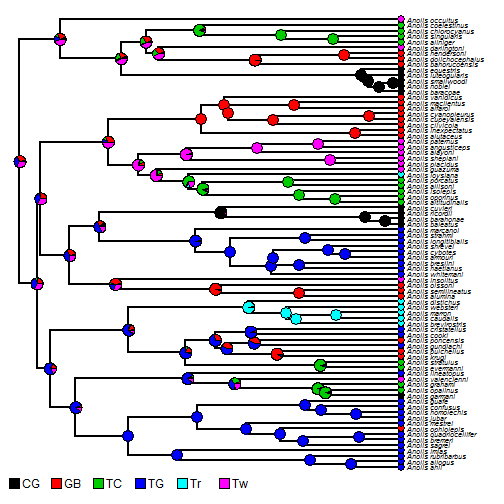This fix can be obtained most easily by installing phytools from GitHub using devtools. In a new R session, run:

``````library(devtools)
install_github("liamrevell/phytools")
``````

That's it.

## Thursday, September 24, 2015

### New version of phytools (0.5-00) submitted to CRAN

I have just submitted a new version of phytools (phytools 0.5-00) to CRAN. Hopefully, it survives the CRAN maintainer scrutiny & posts within the next 24 hours; however it can already be installed from GitHub using devtools:

``````library(devtools)
install_github("liamrevell/phytools",quiet=TRUE)
packageVersion("phytools")
``````
``````##  '0.5.0'
``````

This version of phytools has many changes and new functionality when compared to the previous CRAN version from early July, so it will be hard to be fully comprehensive about the updates; however, among the changes in this CRAN version, users will find:

1. A new method for co-phylogenetic plotting (e.g., 1, 2, 3, 4).

2. A bug fix in `plot.ltt` with a plotted tree overlain that caused the tip labels to be spaced exponentially.

3. An important bug fix in the phytools function to test for differences in the evolutionary correlation between traits in different parts of the tree, based on Revell & Collar (2009; Evolution).

4. A very nice update/improvement to the phytols function (`cladelabels`) for adding clade labels to a plotted tree (here).

5. A function (`markChanges`) to demarcated & extract the timing of changes from a stochastic character map style tree (1, 2, 3, 4).

6. A change in the way object class is checked in phytools that affected the source code of many different phytools function (here).

7. Some changes to `plotSimmap` and `cladelabels` to permit them to be used more effectively with the function `split.plotTree` (here).

8. A major update to the object class of `"phylo"` objects with a mapped discrete character, along with S3 methods for this object class (`print`, `plot`, `summary`, etc.; e.g., 1, 2).

9. An update to the function `treeSlice` to slice the tree at a particular point & return all subtrees (that, among other things, allows this to be done interactively).

10. A fix to the default color scheme used by `phenogram` with a mapped discrete character.

11. An update to the phytools function `phylomorphospace` that allows it to play with ape functions such as `nodelabels` (and thus permits us to plot labels at internal or external nodes of a phylogeny projected into 2D morphospace.

12. A new S3 plotting method for objects of class `"rateshift"` created by the function to estimate the temporal position of a shift in the evolutionary rate for a continuous character through time.

13. A small bug fix in the phytools function `drop.tip.densityMap`.

14. An update to `plotSimmap` to allow the painted color to be split along the vertical edge plotted at a node, for when the character changes state exactly at a node (1, 2).

15. A new S3 `print` methods for objects created by the phytools ancestral state estimation function `fastAnc` (here).

16. An important update to the internal functions used by `make.simmap` to compute the likelihood, as well as the conditional likelihoods at internal nodes. This primarily affects users who are using a non-default prior at the root node of the tree.

17. A new function, `fitMk`, to fit the Mk model. (Functionally similar to `fitDiscrete` in geiger.)

18. A more or less imperceptible update to the function `rerootingMethod`. This basically allows the function to handle polytomies “natively” (i.e., without first having to resolve them, perform calculations using the resolved tree, and then match nodes back to the original tree).

19. An additional estimation method (and a change in the default estimation method) for the phytools function `fitPagel`, which fits the Pagel (1994) model for testing whether the evolution of two binary characters is correlated.

20. An update to the function `rateshift` for testing for temporal shifts in the rate of evolution for a continuous trait that permits much more effective (if not faster) optimization. (Prior versions really could not maximize the likelihood effectively for more than - at best - two shifts on reasonably sized trees.)

21. Finally, some modifications to the phytools function `brownieREML`, and a REML version of the function `rateshift`, which runs much faster than the ML version (here).

Feedback is always welcome on any issues created by these changes. (Of course, I'm always happy to take positive feedback too!)

### Modifications to brownieREML & new REML version of rateshift

I just updated the phytools function `brownieREML`, which implements a REML (restricted maximum likelihood) version of `brownie.lite` which is in turn based on Brian O'Meara et al. (2006; Evolution) method to test for multiple rates of continuous character evolution on the tree, where the branches associated with each rate regime are specified a priori by the user.

(1) Using the function `optimHess` I estimate the variance on the parameter estimates from the negative inverse of the Hessian matrix.

(2) I change some of the names of the elements of the list returned by `brownieREML` to match the corresponding elements from the function `brownie.lite`.

(3) Finally, I added an object class (`"brownieREML"`) and an S3 `print` method to the object returned by `brownieREML`.

Here's a very quick demo of these attributes:

``````library(devtools)
install_github("liamrevell/phytools",quiet=TRUE)
``````
``````library(phytools)
``````
``````## Loading required package: ape
``````
``````## set seed
set.seed(1)
## simulate multiple temporal regimes with different rates:
tree<-pbtree(n=100,scale=100)
mtree<-make.era.map(tree,c(0,75))
x<-sim.rates(mtree,setNames(c(10,1),1:2))
plot(mtree,ftype="off",colors=setNames(c("red","blue"),1:2))
c("fast rate","slow rate")),prompt=FALSE,x=0,y=Ntip(mtree))
``````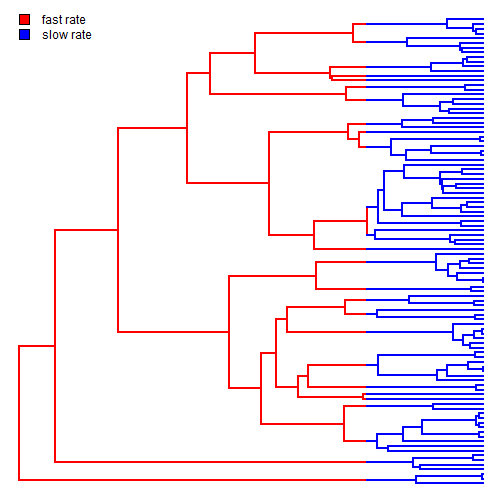``````## fit using brownie.lite
fit.ml<-brownie.lite(mtree,x)
fit.ml
``````
``````## ML single-rate model:
##  s^2 se  a   k   logL
## value    2.7271  0.3856  8.5444  2   -323.1423
##
## ML multi-rate model:
##  s^2(1)  se(1)   s^2(2)  se(2)   a   k   logL
## value    14.2251 4.3892  0.7519  0.1221  8.5015  3   -285.7067
##
## P-value (based on X^2): 0
##
## R thinks it has found the ML solution.
``````
``````## fit using brownieREML
fit.reml<-brownieREML(mtree,x)
fit.reml
``````
``````## REML single-rate model:
##  s^2 se  k   logL
## value    2.7547  0.3915  1   -320.173
##
## REML multi-rate model:
##  s^2(1)  se(1)   s^2(2)  se(2)   k   logL
## value    14.9208 4.691   0.7511  0.1221  2   -281.947
##
## R thinks it has found the REML solution.
``````

The main reason for this update is to permit `brownieREML` to be used internally by `rateshift` for `rateshift(...,method="REML")`.

Here's more or less how that would look (along with the speed-up that results):

``````system.time(
fit.ml<-rateshift(tree,x,method="ML",nrates=2)
)
``````
``````## Optimization progress:
## |..........|
## Done.
``````
``````##    user  system elapsed
##  402.82    0.53  413.34
``````
``````fit.ml
``````
``````## ML 2-rate model:
##  s^2(1)  se(1)   s^2(2)  se(2)   k   logL
## value    13.7226 4.3593  0.7304  0.1196  4   -285.3171
##
## Shift point(s) between regimes (height above root):
##  1|2 se(1|2)
## value    75.6848 1.2073
##
## Model fit using ML.
##
## Frequency of best fit: 0.4
##
## R thinks it has found the ML solution.
``````
``````system.time(
fit.reml<-rateshift(tree,x,method="REML",nrates=2)
)
``````
``````## Optimization progress:
## |..........|
## Done.
``````
``````##    user  system elapsed
##   31.63    0.19   32.15
``````
``````fit.reml
``````
``````## ML 2-rate model:
##  s^2(1)  se(1)   s^2(2)  se(2)   k   logL
## value    14.4349 4.6601  0.7303  0.1196  4   -281.5724
##
## Shift point(s) between regimes (height above root):
##  1|2 se(1|2)
## value    75.6301 1.1231
##
## Model fit using REML.
##
## Frequency of best fit: 0.3
##
## R thinks it has found the REML solution.
``````

So, it is at least a little faster.

## Saturday, September 19, 2015

### The difference between different methods for fitting the Mk model in R: It's all about the prior

There are multiple functions in R to fit the so-called Mk model for discrete character evolution. For instance, there is `ace` in the ape package, `fitDiscrete` in geiger, and now `fitMk` in my package, phytools - which has a lot of similarity to `ace`, but some important differences. This does not even consider the functions of phangorn and diversitree, which can also be used to fit this model.

A casual user may discovery, however, that although these methods all purport to be fitting the same model, parameter estimates and likelihoods often differ ever so slightly (but more than one would expect based on the limits of numerical precision) between methods.

What accounts for this, it turns out, is different implicit or explicit assumptions about the prior probability distribution at the global root of the tree. This is difficult to track down in documentation for any of these functions, although in `fitMk` the assumed prior (`pi`) is reported explicitly (and can be modified), and (as I show below) it is also possible to use `fitDiscrete` to fit the Mk model with any prior.

``````## load packages
library(phytools)
library(geiger)
``````

Next, let's simulate some data under the Mk model:

``````## simulate some data
tree<-pbtree(n=100,scale=1)
Q<-matrix(c(-2,1,1,1,-2,1,1,1,-2),3,3)
rownames(Q)<-colnames(Q)<-letters[1:3]
Q
``````
``````##    a  b  c
## a -2  1  1
## b  1 -2  1
## c  1  1 -2
``````
``````x<-sim.history(tree,Q,anc="a")\$states
``````
``````## Done simulation(s).
``````
``````x
``````
``````##  t53  t54  t89  t90   t9  t10   t6  t11  t24  t25  t12  t44  t62  t67  t74
##  "a"  "a"  "c"  "c"  "a"  "a"  "b"  "b"  "a"  "a"  "a"  "a"  "a"  "b"  "a"
##  t75  t65  t66  t45  t81  t82  t69  t35  t36  t70  t71   t1   t5  t17  t23
##  "a"  "c"  "c"  "a"  "a"  "c"  "a"  "a"  "a"  "b"  "c"  "c"  "a"  "a"  "a"
##  t91  t92  t55  t56  t46  t47  t50  t51  t22  t78  t85  t86  t61  t63  t64
##  "c"  "b"  "c"  "c"  "c"  "c"  "c"  "c"  "c"  "c"  "c"  "c"  "c"  "c"  "c"
##  t18  t95  t96  t13  t42  t43  t93  t94  t99 t100  t15   t4  t40  t41   t2
##  "c"  "b"  "b"  "c"  "c"  "c"  "c"  "c"  "a"  "a"  "b"  "c"  "b"  "b"  "b"
##   t3  t76  t77  t39  t38  t30  t27  t31  t83  t84  t57  t58  t37  t14  t19
##  "b"  "b"  "a"  "a"  "a"  "b"  "a"  "a"  "a"  "a"  "a"  "a"  "c"  "a"  "a"
##  t20   t7  t79  t80  t21   t8  t68  t87  t88  t52  t72  t73  t33  t34  t16
##  "a"  "c"  "b"  "b"  "b"  "a"  "a"  "a"  "a"  "a"  "b"  "b"  "b"  "b"  "b"
##  t26  t28  t29  t48  t49  t97  t98  t32  t59  t60
##  "b"  "c"  "a"  "a"  "a"  "b"  "b"  "b"  "a"  "b"
``````

Now, we can fit each model under the generating model (`model="ER"`):

``````## fit models
fit.phytools<-fitMk(tree,x,model="ER",pi="equal") ## flat root
fit.phytools
``````
``````## Object of class "fitMk".
##
## Fitted (or set) value of Q:
##           a         b         c
## a -1.773072  0.886536  0.886536
## b  0.886536 -1.773072  0.886536
## c  0.886536  0.886536 -1.773072
##
## Fitted (or set) value of pi:
##         a         b         c
## 0.3333333 0.3333333 0.3333333
##
## Log-likelihood: -86.449618
``````
``````fit.ape<-ace(x,tree,type="discrete",model="ER")
fit.ape
``````
``````##
##     Ancestral Character Estimation
##
## Call: ace(x = x, phy = tree, type = "discrete", model = "ER")
##
##     Log-likelihood: -85.35101
##
## Rate index matrix:
##   a b c
## a . 1 1
## b 1 . 1
## c 1 1 .
##
## Parameter estimates:
##  rate index estimate std-err
##           1   0.8865  0.1524
##
## Scaled likelihoods at the root (type '...\$lik.anc' to get them for all nodes):
##         a         b         c
## 0.4114971 0.3071304 0.2813725
``````
``````fit.geiger<-fitDiscrete(tree,x,model="ER")
fit.geiger
``````
``````## GEIGER-fitted comparative model of discrete data
##  fitted Q matrix:
##                a          b          c
##     a -1.7668479  0.8834239  0.8834239
##     b  0.8834239 -1.7668479  0.8834239
##     c  0.8834239  0.8834239 -1.7668479
##
##  model summary:
##  log-likelihood = -86.421424
##  AIC = 174.842847
##  AICc = 174.883663
##  free parameters = 1
##
## Convergence diagnostics:
##  optimization iterations = 100
##  failed iterations = 0
##  frequency of best fit = 1.00
##
##  object summary:
##  'lik' -- likelihood function
##  'bnd' -- bounds for likelihood search
##  'res' -- optimization iteration summary
##  'opt' -- maximum likelihood parameter estimates
``````

We can see that each is slightly different - either in the fitted model, or the likelihood, or both!

To show that this is due to different priors on the root node, we can change the prior using the object created by `fitDiscrete`. This is not super-straightforward, but kind of neat in the end. (There may be some way to do this within a call of `fitDiscrete`, but I was not able to do that.)

``````## the likelihood function
lik<-fit.geiger\$lik
## optimize it using a flat/"equal" prior
fit.flat<-optimize(lik,c(1e-8,1000),root=ROOT.FLAT,maximum=TRUE)
fit.flat
``````
``````## \$maximum
##  0.8865379
##
## \$objective
##  -86.44962
``````

If we compare this rate parameter to `fitMk` or `ace`, or the likelihood to `fitMk`, we should see that it is equal. Cool.

### Update to rateshift method that results in much better optimization for models with multiple shifts

I have been working on the phytools function `rateshift`, for locating one or multiple temporal shifts in the rate of evolution for a continuous character on the tree.

This function has nice `print` and `plot` methods, and would seem to be quite nice - however it suffers from one fairly critical problem which is that it doesn't really work. That is to say, it frequently fails to find the MLE solution.

I have put a little effort into overhauling the guts of this function now; with the main and most critical update being that the function now uses the phytools function `brownie.lite` to find the MLE rates conditioned on a given set of shift points; rather than simultaneously optimizing rates & shift points. This effectively halves the dimensionality of the optimization, and this seems to have had a very nice effect on performance (although there is still the chance of being stuck on local optima, as we'll see below).

``````library(devtools)
install_github("liamrevell/phytools",quiet=TRUE)
library(phytools)
``````
``````## Loading required package: ape
``````

Now, we can start by simulating a tree and data in which there are two shift points, and thus three Brownian rates:

``````set.seed(99)
tree<-pbtree(n=50,scale=100)
x<-sim.rates(tree<-make.era.map(tree,c(0,60,85)),
setNames(c(5,20,1),1:3))
## these are our simulated regimes:
plot(tree,setNames(c("purple","red","blue"),1:3),fsize=0.8,ftype="i",
mar=c(3.1,0.1,0.1,0.1))
axis(1)
``````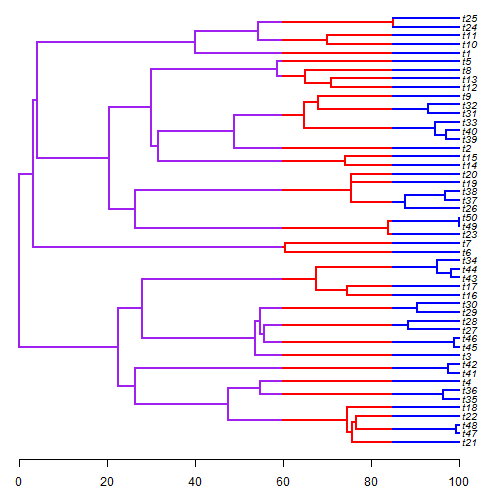Let's fit one, two, three, and four rate models to these data:

``````fit1<-rateshift(tree,x,nrates=1,niter=10)
``````
``````## Optimization progress:
## |..........|
## Done.
``````
``````fit1
``````
``````## ML 1-rate model:
##  s^2(1)  se(1)   k   logL
## value    7.4779  1.4956  2   -206.1193
##
## This is a one-rate model.
##
## Frequency of best fit: 1
##
## R thinks it has found the ML solution.
``````
``````fit2<-rateshift(tree,x,nrates=2,niter=10)
``````
``````## Optimization progress:
## |..........|
## Done.
``````
``````fit2
``````
``````## ML 2-rate model:
##  s^2(1)  se(1)   s^2(2)  se(2)   k   logL
## value    13.8711 3.3579  0.5263  0.2124  4   -193.311
##
## Shift point(s) between regimes (height above root):
##  1|2 se(1|2)
## value    88.4271 0.0436
##
## Frequency of best fit: 0.9
##
## R thinks it has found the ML solution.
``````
``````fit3<-rateshift(tree,x,nrates=3,niter=10)
``````
``````## Optimization progress:
## |..........|
## Done.
``````
``````fit3
``````
``````## ML 3-rate model:
##  s^2(1)  se(1)   s^2(2)  se(2)   s^2(3)  se(3)   k   logL
## value    7e-04   NaN 16.5352 4.7994  0.5158  0.2057  6   -190.4577
##
## Shift point(s) between regimes (height above root):
##  1|2 se(1|2) 2|3 se(2|3)
## value    48.8951 10.6356 88.4271 0.0387
##
## Frequency of best fit: 0.7
##
## R thinks it has found the ML solution.
``````
``````fit4<-rateshift(tree,x,nrates=4,niter=10)
``````
``````## Optimization progress:
## |..........|
## Done.
``````
``````fit4
``````
``````## ML 4-rate model:
##  s^2(1)  se(1)   s^2(2)  se(2)   s^2(3)  se(3)   s^2(4)  se(4)   k   logL
## value    7e-04   NaN 833.039 NaN 5.0879  2.2729  0.5437  0.2229  8   -188.5406
##
## Shift point(s) between regimes (height above root):
##  1|2 se(1|2) 2|3 se(2|3) 3|4 se(3|4)
## value    68.2645 NaN 69.0033 NaN 90.5243 0.07
##
## Frequency of best fit: 0.2
##
## R thinks it has found the ML solution.
``````
``````AIC(fit1,fit2,fit3,fit4)
``````
``````##      df      AIC
## fit1  2 416.2387
## fit2  4 394.6221
## fit3  6 392.9153
## fit4  8 393.0813
``````

We can plot our best model, in this case the generating, three-rate model:

``````plot(fit3,fsize=0.8)
``````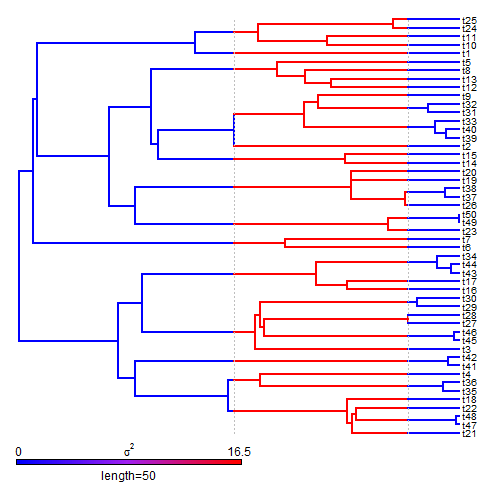Finally, I mentioned that it is possible to get trapped on local optima. This is true, somewhat surprisingly, even for the relatively simple, two-rate model. We can see why by visualizing the likelihood surface across the length of the tree:

``````shift<-1:99/100*max(nodeHeights(tree))
logL<-sapply(shift,function(s,tree,x) logLik(rateshift(tree,x,fixed.shift=s,
quiet=TRUE)),tree=tree,x=x)
plot(shift,logL,type="l",lwd=2)
plotTree(tree,color=rgb(0,0,1,0.25),ftype="off",mar=c(5.1,4.1,4.1,2.1),
``````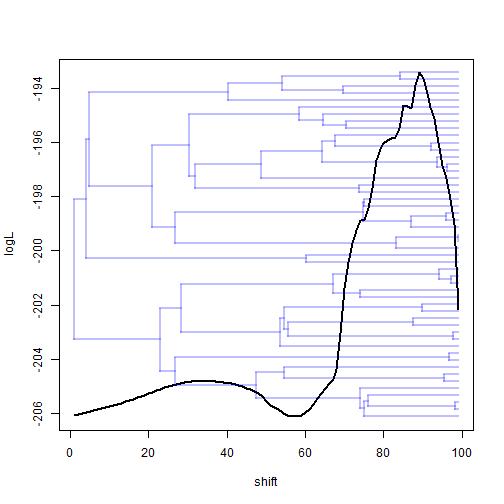We can also do this in two dimensions for the three-rate model:

``````shift<-seq(2,98,by=2)/100*max(nodeHeights(tree))
logL<-sapply(shift,function(s1,s2,tree,x)
sapply(s2,function(s2,s1,tree,x)
logLik(rateshift(tree,x,fixed.shift=if(s1!=s2) c(s1,s2) else s1,
quiet=TRUE)),s1=s1,tree=tree,x=x),s2=shift,tree=tree,x=x)
image(shift,shift,logL,col=gray.colors(60,0,1),xlab="shift 1 (or 2)",
ylab="shift 2 (or 1)")
points(fit3\$shift,fit3\$shift,pch=4) ## MLE
points(fit3\$shift,fit3\$shift,pch=4)
``````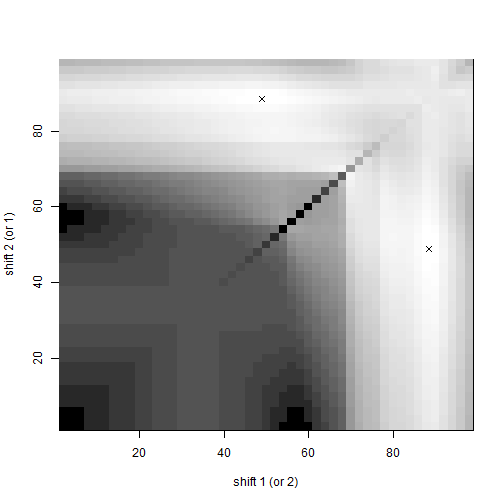Or, alternatively:

``````library(lattice)
wireframe(logL,row.values=shift,column.values=shift,xlab="shift 1 (or 2)",
ylab="shift 2 (or 1)",colorkey=TRUE,drape=TRUE)
``````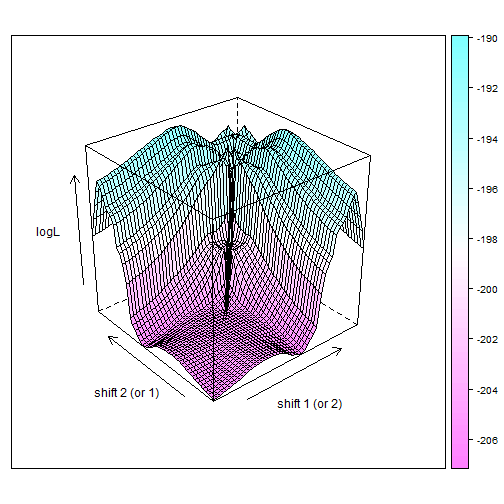Interesting!